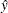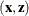# The TPSPLINE Procedure

### SCORE Statement

SCORE DATA=SAS-data-set OUT=SAS-data-set <keyword …  keyword> ;

The SCORE statement calculates predicted statistics for a new data set. If you have multiple data sets to predict, you can specify multiple SCORE statements. You must use a SCORE statement for each data set.

You can request diagnostic measures that are calculated for each observation in the SCORE data set. The new data set contains all the variables in the SCORE data set in addition to the requested variables. If no keyword is present, the data set contains only the predicted values.

The following keywords must be specified in the SCORE statement:

DATA=SAS-data-set

specifies the input SAS data set that contains the smoothing variablesand regression variables. The predicted response () value is computed for eachpair. The data set must include all independent variables specified in the MODEL statement.

OUT=SAS-data-set

specifies the name of the SAS data set to contain the predictions.

keyword

specifies the statistics to include in the output data set for the current SCORE statement. The names of the new variables that contain the statistics are formed by using a prefix of one or more characters to identify the statistic, followed by an underscore (_), followed by the dependent variable name. The keywords and the statistics they represent are as follows:

PRED

predicted values

STD

standard error of the mean predicted value

UCLM

upper limit of the Bayesian confidence interval for the expected value of the dependent variables. By default, PROC TPSPLINE computes 95% confidence limits.

LCLM

lower limit of the Bayesian confidence interval for the expected value of the dependent variables. By default, PROC TPSPLINE computes 95% confidence limits.